Get instant live expert help with Excel or Google Sheets“My Excelchat expert helped me in less than 20 minutes, saving me what would have been 5 hours of work!”

#### Post your problem and you’ll get Expert help in seconds.

Your message must be at least 40 characters
Our professional Expert are available now. Your privacy is guaranteed.

# How to Highlight the 3 Smallest Values with Criteria in ExcelRead time: 15 minutes

While working with Excel, we are able to highlight values based on a condition or criteria by using Conditional Formatting.  This step by step tutorial will assist all levels of Excel users in highlighting the three smallest values with criteria by using the AND, SMALL and IF functions.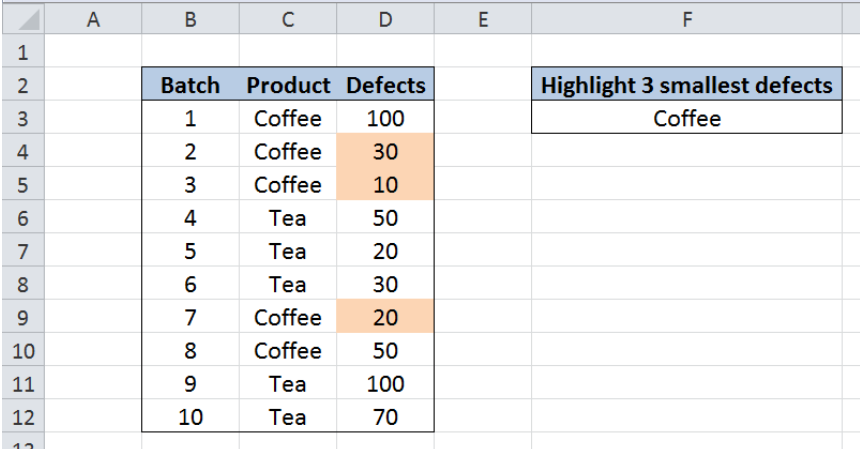Figure 1. Final result: Highlight 3 smallest values with criteria

Final formula:  `=AND(C3=\$F\$3,\$D3<=SMALL(IF(\$C\$3:\$C\$12=\$F\$3,\$D\$3:\$D\$12),3))`

## Syntax of AND Function

AND function evaluates all logical tests and returns a TRUE if all arguments are TRUE; FALSE if one or more arguments is FALSE

`=AND(logical1, [logical2], ...)`

• logical1–  the first condition that we want to test
• only logical1 is required; succeeding conditions are optional

## Syntax of SMALL function

SMALL returns the k-th smallest value in a data set

`=SMALL(array, k)`

• array – An array or range for which we want to determine the k-th smallest value
• k  – the position of the value in the range or data set that we want to return
• SMALL returns the #NUM! error value when the array is empty, if k≤ 0,or if k exceeds the number of data points

## Syntax of IF Function

IF function evaluates a given logical test and returns a TRUE or a FALSE

`=IF(logical_test, [value_if_true], [value_if_false])`

• The arguments “value_if_true” and “value_if_false” are optional.  If left blank, the function will return TRUE if the logical test is met, and FALSE if otherwise.

## Setting up Our Data

Our table contains three columns: Batch (column B), Product (column C) and Defects (column D).  In cell F3, we enter the criteria “Coffee”. We want to highlight the three smallest defects for the product Coffee.  We can do this through Conditional Formatting.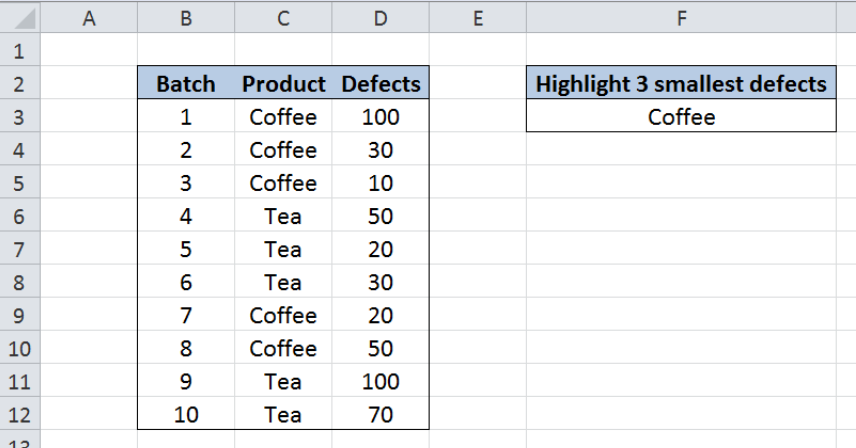Figure 2. Sample data to highlight 3 smallest values with criteria

## Highlight the 3 smallest defects for Coffee

Highlighting values based on a given criteria or condition can be done by using Conditional Formatting, and entering a formula that determines which cells we want to format.  Let us follow these steps:

Step 1.  Select the cells we want to highlight; In this case, select D3:D12

Step 2. Click the Home tab, then the Conditional Formatting Menu and select “New Rule”. The New Formatting Rule dialog box will pop up.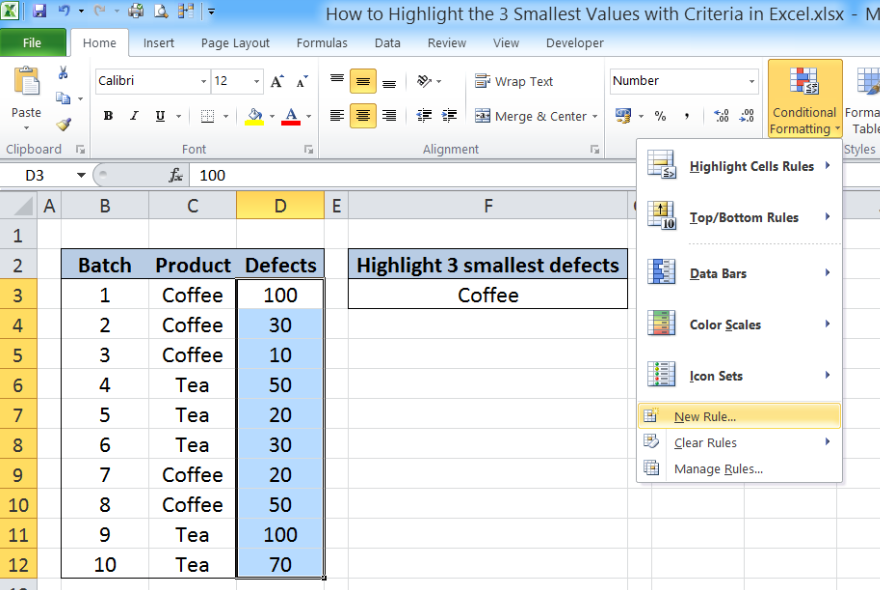Figure 3.  Creation of a new rule in conditional formatting

Step 3. Select the Rule Type “Use a formula to determine which cells to format” and enter this formula in the dialog box :

`=AND(C3=\$F\$3,\$D3<=SMALL(IF(\$C\$3:\$C\$12=\$F\$3,\$D\$3:\$D\$12),3))`

Our formula serves as the condition or rule that will trigger the conditional formatting.  The dollar signs “\$” fix specific cells that will enable our formula to function properly for all cells in D3:D12.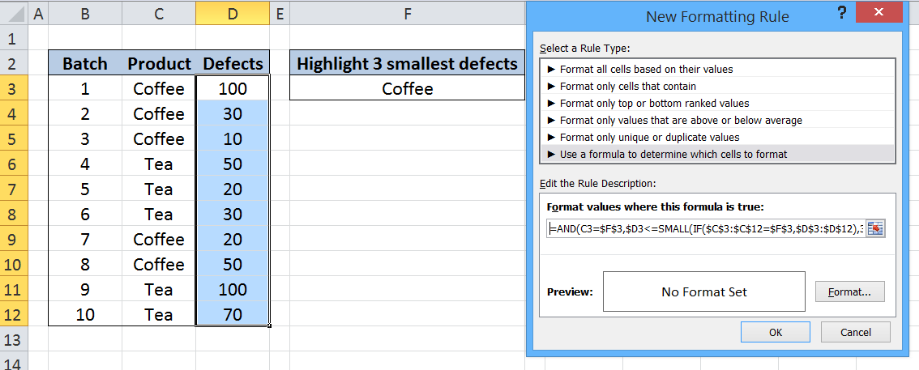Figure 4.  Entering the formula as a condition or formatting rule

The cells in column D will be highlighted when the formula returns TRUE.  Our AND formula has two criteria. First, the cell in column C must be equal in value to cell F3 or “Coffee”.  Second, the cell in column D must be less than or equal to the third smallest value of the defects for Coffee.

## How the formula works

The third smallest value for Coffee defects is determined by the SMALL function.  Our array for the SMALL function is the IF function, while the value for k is 3.  This means that we want to find the third smallest value from the array resulting from the IF function.

The IF function has the condition `\$C\$3:\$C\$12=\$F\$3` which means that we want to find only the values in column C that are equal to F3 or Coffee. The logical test returns the array:

`{TRUE;TRUE;TRUE;FALSE;FALSE;FALSE;TRUE;TRUE``;FALSE;FALSE}`

where the TRUE values correspond to the positions of Coffee in column C.

For every logical TRUE, the IF function returns the corresponding value in column D.  Applying the IF function to the array formula results to: `{``100;30;10;FALSE;FALSE;FALSE;20;50;FALSE;FALSE} `

The SMALL function returns the third smallest value in the array, which is 30. Finally, our formula highlights the cells in column C when the value in column B is “Coffee” and the value in column C is less than or equal to 30.

To change the format, let us proceed to the next step.

Step 4. Click “Format” and then decide on the new format to apply to the cells in column C.  We can change the font, borders or fill the cells with different colors.

## Example

Select “Fill” and choose Orange, Accent 6, Lighter 60% and click OK.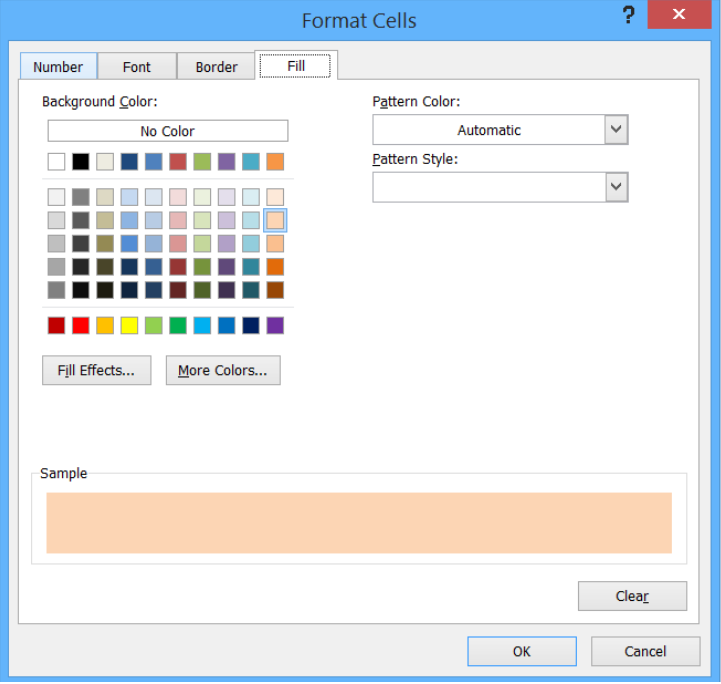Figure 5.  Selection of the format to use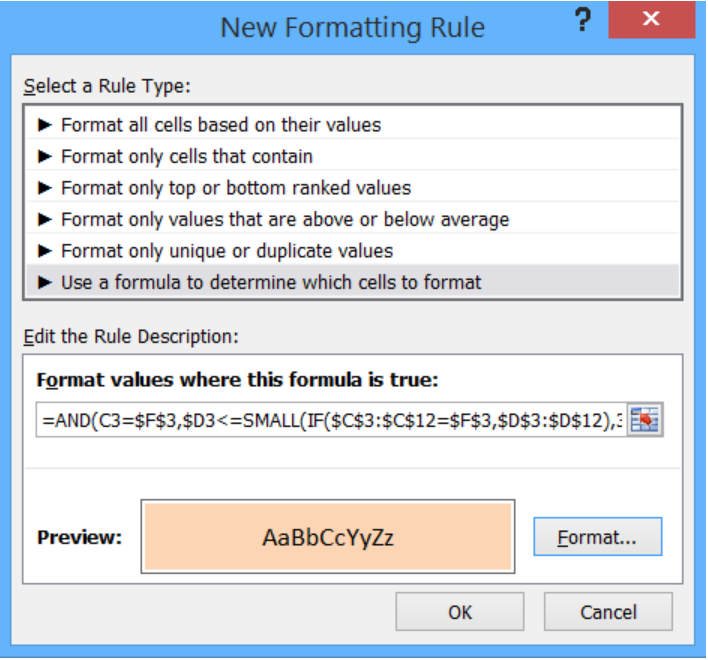Figure 6.  Completion of the new formatting rule with formula and format

This rule highlights the cells that satisfy the conditions of C3=F3 and D3<=30.  As a result, the cells D4, D5 and D9 with values 30, 10 and 20 are highlighted as shown below.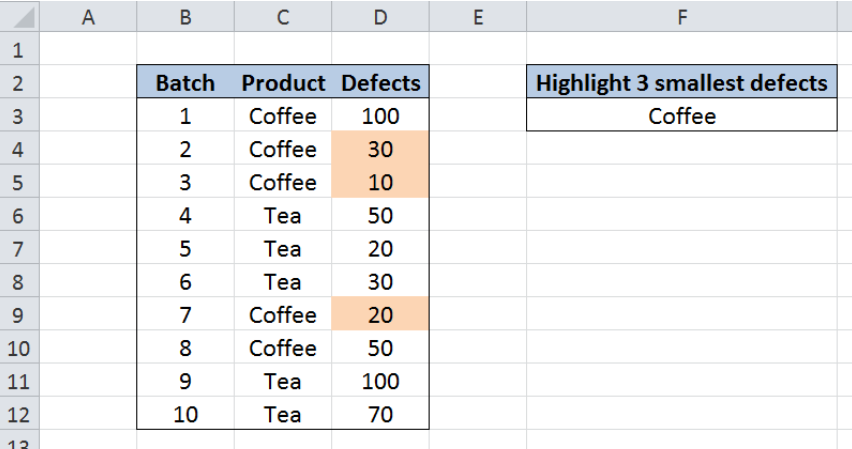Figure 7.  Output: Highlight 3 smallest values with criteria

Most of the time, the problem you will need to solve will be more complex than a simple application of a formula or function. If you want to save hours of research and frustration, try our live Excelchat service! Our Excel Experts are available 24/7 to answer any Excel question you may have. We guarantee a connection within 30 seconds and a customized solution within 20 minutes.

Are you still looking for help with Conditional Formatting? View our comprehensive round-up of Conditional Formatting tutorials here.

### Did this post not answer your question? Get a solution from connecting with the expert.Another blog reader asked this question today on Excelchat:
Solution examplesI have a list or people who are ranked in numbers from 3 to 6 I need to recognize the contents of each multiple cells and create an equivalent letter. for that value into another column. e.g. Cell E1 = 3 to show in new cell that row (H1) the letter "C" I have multiple rows with different values in column "E" Can this be done?
Solved by I. J. in 30 minsCould you help me by explaining the IF function?
Solved by G. L. in 24 minsYes hello Im looking for help on conditional formatting I currently have a conditional format for D9>D32 and so on from D9:S20. I need to add an if statement that references cell D4 if the first four characters are 1358 for the rule to apply can you please assist?
Solved by Z. U. in 20 minsI want to display a range of cells if they meet a specific criteria. I want to display a name IF it does not equal one of three options AND there are any numbers contained in a range of cells
Solved by G. F. in 60 minsA2=50 A3=46 A4=39 =IF(A2>=48,100) =IF(AND(A3<=47,A3>=41),120) =IF(A4<=40,A4*3) Individually this logic formula works with the desired value result. When I nested the IF's the only the first logic statement gave me a value result the other logic statements gave me a "False". Here is the nested formula I created. =IF(A2<=40,A2*3,IF(AND(A2<=47,A2>=41,120),IF(A2>=48,100)))
Solved by C. C. in 11 mins## Subscribe to Excelchat.coAnother blog reader asked this question today on Excelchat: